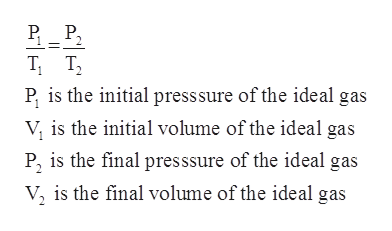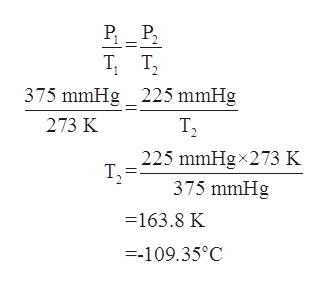# Calculate the final Celcius temperature of nitrogen dioxide gas if 1.95L of the gas at 0 degrees Celsius and 375 mmHg is cooled until the pressure is 225 mmHg,  assuming no volume change.

Question
42 views

Calculate the final Celcius temperature of nitrogen dioxide gas if 1.95L of the gas at 0 degrees Celsius and 375 mmHg is cooled until the pressure is 225 mmHg,  assuming no volume change.

check_circle

star
star
star
star
star
1 Rating
Step 1

The Gay-Lussac’s law is an ideal gas law according to which the pressure of an ideal gas is directly proportional to its absolute temperature (in Kelvin) provided that the volume remains constant. The mathematical formula is as follows:help_outlineImage TranscriptioncloseP, P, T T, P, is the initial presssure of the ideal gas V, is the initial volume of the ideal gas P, is the final presssure of the ideal gas V, is the final volume of the ideal gas || fullscreen
Step 2

In the given problem, the initial pressure of the nitrogen dioxide gas is given to be 375 mmHg.

The initial temperature of the gas is given to be 0oC=27...help_outlineImage TranscriptioncloseP_ P, T T, 1. 375 mmHg _ 225 mmHg 273 K T2 225 mmHgx273 K T, 375 mmHg =163.8 K =-109.35°C fullscreen

### Want to see the full answer?

See Solution

#### Want to see this answer and more?

Solutions are written by subject experts who are available 24/7. Questions are typically answered within 1 hour.*

See Solution
*Response times may vary by subject and question.
Tagged in

### Physical Chemistry